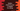# Python tutorial to check if a user is eligible for voting or not## Python tutorial to check if a user is eligible for voting or not :

In this python tutorial, we will learn how to check if a user is eligible for voting or not. The program will take the age as an input from the user, check the eligibility and then print out the result.

In this program, we will assume that a person can vote if his/her age is more than 18 years. This is a beginner-level python program. You will learn how to check a condition in python with this tutorial.

This program will give you a basic understanding of the ternary if-else condition and how to read user input values. Before moving to the main program, let’s quickly take a look at the ternary if-else condition :

### Definition :

The ternary if-else condition is also called conditional expression in Python. They were introduced in Python 2.4. The ternary if-else condition is defined as below.

``code_if_true if condition else code_if_false``

Using it, we can quickly check a condition instead of writing multiple lines of code. Based on the condition, it will return us the required value.

In the definition above, it will first check if ‘condition’ is True or False. If it is True, the final value will be codeiftrue , else it will be codeiffalse.

A four lines of if-else code can be converted to one line using ternary if-else. In the below program, we will explain to you how it actually works. Let’s take a look at the program first :

### Python program :

``````age = int(input("Enter your age: "))

print("You are eligible for voting" if age > 18 else "You are not eligible for voting")``````

### Output :

As you can see that our program is only of two lines. Let’s take a look at the steps of the program one by one :

1. First of all, we are reading the input from the user using the input() method. This method returns a string, so we are using int(input()) to convert the input to an integer.
2. Next, we are checking if the value is greater than 18 or not. This is known as a ternary conditional operator in python. If you are familiar with C language, then you may have seen the “condition ? statement : statement ” operator. This is the same.

So, it will check if the user is eligible or not for voting and print out the result accordingly.

You can also use the ‘if-else’ block to achieve the same result. But ternary if-else will fit more for this scenario.

Ternary if-else is an important python condition checker. If you want to check any condition with only one line, you can use ternary if-else. Else, you can use the normal if-else. It makes the code more compact.

### Conclusion :

This program has taught you how to use the ternary conditional operator in python and how to read a user input number. Try to run the program on your side and drop a comment below if you have any queries.